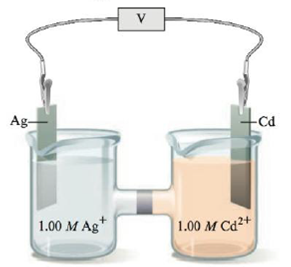Chapter 18, Problem 153CP

Chapter
Section
Textbook Problem

# Consider the following galvanic cell:A 15 0-mole sample of NH  is added to the Ag compartment (assume 1.00 L of total solution after the addition). The silver ion reacts with ammonia to form complex ions as shown: Ag + ( a q )   +   NH 3 ( a q )   ⇌ AgNH 3 + ( a q ) K 1 = 2.1 × 10 3 AgNH 3 + ( a q )   +   NH 3 ( a q )   ⇌ Ag ( NH 3 ) 2 + ( a q ) K 2 = 8.2 × 10 3 Calculate the cell potential after the addition of 15.0 moles of NH3.

Interpretation Introduction

Interpretation: The cell potential after the addition of 15.0 moles of NH3 to the given cell compartment is to be calculated.

Concept introduction: Cell potential is defined as the measure of energy per unit charge that is the potential difference in an electrochemical cell containing two half cells. The cell potential in an electrochemical cell is measured by using Nernst equation.

To determine: The cell potential after the addition of 15.0 moles of NH3 to the Ag compartment.

Explanation

Explanation

The standard cell potential of the given cell is 1.20 V_ .

The half cell reactions of the given cell are,

Anode:    Cd(s)Cd2++2e                   E1ο=0.40V (1)

Cathode:   (Ag+(aq)+eAg(s))×2      E2ο=0.80V (2)

Where,

• E1ο is the standard electrode potential of equation (1).
• E2ο is the standard electrode potential of equation (2).

The resultant cell reaction obtained by adding equation (1) and (2) is,

Cell:  2Ag+(aq)+Cd(s)Cd2+(aq)+2Ag(s) (3)

The standard electrode potential of equation (3) is calculated by the formula,

Ecellο=EcathodeοEanodeο

0r, E3ο=E2οE1ο

Where,

• E3ο is the standard electrode potential of equation (3).

Substitute the values of E1ο and E2ο in the above formula.

E3ο=0.80 V(0.40 V)=1.20V

Hence, the standard cell potential of the given cell is 1.20 V_ .

The formation constant (K) for the reaction of formation of complex ion is 1.7×107_ .

The reactions for the formation of complex ion are given as,

Ag+(aq)+NH3(aq)Ag(NH3)(aq)               K1=2.1×103 (4)

Ag(NH3)+(aq)+NH3(aq)Ag(NH3)2+(aq)   K2=8.2×103 (5)

Where,

• K1 is the equilibrium constant of equation (4).
• K2 is the equilibrium constant of equation (5).

The resultant cell reaction obtained by adding equation (1) and (2) is,

Ag+(aq)+2NH3(aq)Ag(NH3)2+(aq) (6)

The formation constant of equation (6) is calculated by the formula,

K=K1×K2

Where,

• K is formation constant of equation (6).

Substitute the values of K1 and K2 in the above expression to calculate the formation constant.

K=K1×K2=(2.1×103)(8.2×103)=1.7×107_

Hence, the equilibrium constant (K) for the reaction of formation of complex ion is 1.7×107_ .

As the value of (K) is large, the reaction goes to completion and backward reaction take place.

The equilibrium concentration of silver ion [Ag+] is 3.5×10-10_ .

Given

Moles of NH3 is 15

### Still sussing out bartleby?

Check out a sample textbook solution.

See a sample solution

#### The Solution to Your Study Problems

Bartleby provides explanations to thousands of textbook problems written by our experts, many with advanced degrees!

Get Started

#### Find more solutions based on key concepts